Numbers to mass

via the formula mass

Ca =40, Cl =35.5, S =32, N =14, O =16, H =1.

 Compound Number Mole Mass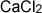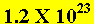Mole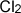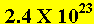Mole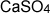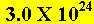Mole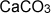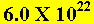Mole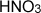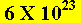Mole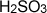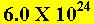Mole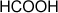Mole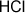Mole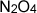Mole

Calculate the mass of 2 X 1023 water molecules.
Solution

How many molecules of sulfuric acid (H2SO4) are present in a 9.8 gram sample of sulfuric acid?

Solution

Home